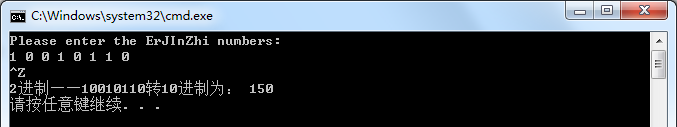• 介绍了C++ 十进制转换为二进制的实例代码，有需要的朋友可以参考一下
• //十进制转换为二进制 #include #include #include #include using namespace std; string s; int main(int argc,char * argv[]) { int n; while(cin>>n) { s=""; for(int a = n; a ;a = a/2
//十进制转换为二进制
#include <iostream>
#include <fstream>
#include <string>
#include <algorithm>
using namespace std;
string s;
int main(int argc,char * argv[])
{
int n;
while(cin>>n)
{
s="";
for(int a = n; a ;a = a/2)
{
//二进制只有两个基数，所以转换起来比较方便，若是转换为其他进制可以使用switch结构
s=s+(a%2?'1':'0');
}
//字符串反转
//s.begin() 获得字符串的开始位置
//s.end() 获得字符串的结尾位置
std::reverse(s.begin(),s.end());

cout<<s<<endl;
//c_str() 将string类型的字符创转化为char*
//const char *sss=s.c_str();
//cout.width(11);
//cout<<n<<(n<0?"-->-":"-->")<<sss<<"\n";

}
return 0;
}

展开全文• 十进制转换为十六进制，也就是 采用 除k取余法 ，直接让10处以16，再对 数据如果小于10和大于10进行处理，代码如下： #include<iostream> #include<string> using namespace std; int main() { ...
十进制转换为十六进制，也就是 采用 除k取余法 ，直接让10处以16，再对 数据如果小于10和大于10进行处理，代码如下：

#include<iostream>
#include<string>
using namespace std;
int main()
{
long a;//输入的数
int y=0;//循环中的余数
string s="";//输出的结果
cin>>a;
if(a==0)//0比较特殊，单独处理
{
cout<<0;
return 0;
}

while(a>0)//大于0的数
{
y=a%16;  //求余
if(y<10)   //小于10的余数
s=char('0'+y)+s;  //利用字符的ascll码在字符串前面拼接
else
s=char('A'-10+y)+s;  //大于9的余数用ABCDE表示
a=a/16;
}
cout<<s;
return 0;
}

十六进制转换为10进制，采取的是 乘以16
#include<iostream>
#include<string>
#include<math.h>
using namespace std;

int main()
{
string s;
int i=0,count,sum;
while(getline(cin,s))
{
int count=s.length();
sum=0;
for(i=count-1;i>=0;i--)//从十六进制个位开始，每位都转换成十进制
{
if(s[i]>='0'&&s[i]<='9')//数字字符的转换
{
sum+=(s[i]-48)*pow(16,count-i-1);
}
else if(s[i]>='A'&&s[i]<='F')//字母字符的转换
{
sum+=(s[i]-55)*pow(16,count-i-1);
}
}
cout<<sum;
}
}  

十进制 转换为 二进制，采取的是 除2取余法，其中把 2 换成其他小于10的数，可以变成相应的 其他进制

#include<iostream>

using namespace std;

int main(){
int a;
cin>>a;
int result=0;
int temp=a;
int j=1;
while(temp){
result=result+j*(temp%2);
temp=temp/2;
j=j*10;
}

cout<<result<<endl;

return 0;
} 

展开全文16进制
• C++实现十进制转换二进制 二进制转换十进制 C++实现二进制转换十进制 十进制与二进制之间的转换 十进制转换二进制 十进制对2整除，得到的余数的倒序即转换而成的二进制 十进制转换二进制 C++实现十进制...

欢迎关注原创公众号 【计算机视觉联盟】，回复 【西瓜书手推笔记】 可获取我的机器学习纯手推笔记！
直达笔记地址：机器学习手推笔记（GitHub地址）

目录
十进制与二进制之间的转换
十进制转换二进制
C++实现十进制转换二进制
二进制转换十进制
C++实现二进制转换十进制

十进制与二进制之间的转换
十进制转换二进制
十进制对2整除，得到的余数的倒序即为转换而成的二进制

十进制转换二进制

C++实现十进制转换二进制
主函数main.cpp为：
#include<iostream>
#include<fstream>
#include<string>
#include<vector>
#include<cmath>
using namespace std;
int main()
{

int N;
cout << "Please enter the number: ";
cin >> N;
cout << endl;

//十进制转二进制的声明和调用
void  convert_10_to_2(int num);
convert_10_to_2(N);
}
十进制转换为二进制函数为：
#include<iostream>
#include<fstream>
#include<string>
#include<vector>
#include<cmath>
using namespace std;
void convert_10_to_2(int num)
{
vector<int> ivec;
int Num = num;  //存储原数据
//对原数据循环求除以2的余数,并存储在vector对象中
while (num != 0)
{
ivec.push_back(num % 2);
num = num / 2;
}

//对求得的余数倒序排序并存储在vector中，求得二进制
vector<int>::size_type sz = ivec.size();
vector<int> ivec2;
for (vector<int>::size_type index = 0; index != sz; ++index)
ivec2.push_back(ivec[sz - 1 - index]);

//输出十进制转换为二进制的数
cout <<Num  << "十进制转二进制后为：";
for (auto item : ivec2)
cout << item;
cout << endl<<endl;
}
运行结果为：二进制转换十进制
将二进制从右往左从零次幂开始乘以二进制数并求和

二进制转换为十进制

C++实现二进制转换十进制
C++主函数为：
#include<iostream>
#include<fstream>
#include<string>
#include<vector>
#include<cmath>
using namespace std;
int main()
{
double convert_2_to_10();
convert_2_to_10();
return 0;
}
二进制转十进制函数为：
#include<iostream>
#include<fstream>
#include<string>
#include<vector>
using namespace std;
double convert_2_to_10()
{
//输入二进制数并存储在vector变量ivec2中
cout << "Please enter the ErJInZhi numbers:  " << endl;
int n;
vector<int> ivec2;
while (cin >> n)
{
ivec2.push_back(n);
}

//二进制转十进制计算
double sum = 0, times;
vector<int>::size_type sz = ivec2.size();
for (vector<int>::size_type index = 0; index != sz; ++index)
{
times = pow(2, sz - 1 - index);
sum += ivec2[index] * times;
}

//输出转化后的结果
cout << "2进制——";
for (vector<int>::size_type index = 0; index != sz; ++index)
cout << ivec2[index];
cout << "转10进制为： ";
cout << sum << endl;
return 0;
}
运行结果：（输入二进制时要以空格区分）展开全文• 主要介绍了C语言用栈实现十进制转换为二进制的方法,结合实例形式分析了C语言栈的定义及进制转换使用技巧,需要的朋友可以参考下
• 使用C++语言实现十进制转换为二进制的运算方法，可正负互转
• C++十进制转换为二进制表示的算法C++十进制转换为二进制表示的算法完整源码(定义，实现，main函数测试） C++十进制转换为二进制表示的算法完整源码(定义，实现，main函数测试） #include <iostream> ...


C++十进制数转换为二进制表示的算法

C++十进制数转换为二进制表示的算法完整源码(定义，实现，main函数测试）

C++十进制数转换为二进制表示的算法完整源码(定义，实现，main函数测试）
#include <iostream>
void method1(int number) {

int remainder, binary = 0, var = 
展开全文• c++ 二进制转换十进制 double power(double x,int n) { double v=1; while(n--) { v=v*x; } return v; }
• 使用C++语言实现十进制转换为二进制的运算方法，可正负互转，解决了上次输出顺序错误的问题
• 十进制二进制（10 分） 输入一个正整数 a（int 型范围内） 编写递归函数输出其二进制形式。 #include<iostream> #include<cmath> using namespace std; void f(int a){ if(a){ f(a/2); cout<<...
• 此文档十进制小数和二进制小数相互转换》，让C++初学者更好的理解进制转换【独家出版，未经允许，禁止侵权】编程
• 十进制数转换为二进制C++前言一、十进制转换为二进制的数学算法二、代码实现1.设计转换函数transfer2.完整代码总结 前言 这篇文章和大家分享一下我个人对十进制数转换为二进制数的想法，目前暂时更新只整数十...算法 编程语言 c语言
• 本文给大家介绍的是一则使用C++实现读入二进制数并转换为十进制输出的代码，实现起来其实非常简单，C++本身就提供了二进制类库的，大家看代码吧，简单又实用。
• 已知一个只包含 0 和 1 的二进制数，长度不大于 10 ，将其转换为十进制并输出。 输入描述 输入一个二进制整数n，其长度大于0且不大于10 输出描述 输出转换后的十进制数， 占一行 样例输入 110 样例...
• C语言-顺序栈实现十进制转换为二进制-八进制-十六进制
• "请输入需要转换十进制数："; cin >> n; int r; int i, j=0; //对十进制数进行除以取余并以此存放到列表中 for ( i = 0;n > 0; i++) { r[i] = n % 2; n = n / 2; j++; } .
• 输出n行，分别对应输入的十进制数。 样例输入 2 11 110 样例输出 3 6 c++： #include <iostream> #include <string> using namespace std; int strToNum(string); int pow(int); void printArr...算法 编程语言
• 数据结构，递归实现十进制数转二进制数，C++实现，可多次输入数数据结构
• C++中，默认状态下，数据按十进制输入输出。...但是二进制没有默认的输出格式，需要自己写函数进行转换。 输入整数n , 则在C++中cout<<hex<<n; cout<<oct<<n; cout<<dec&l...
• 输入-一个十进制的整数，输出该数的十六进制表示。 输入 输入数据有多组，第一个数表示数据的组数n. 然后是n个十进制数 输出 输出n行，每行一个对应的十六进制数。其中大于等于10的数字用大写英文字母ABCDEF...
• 其他转换请参考博文： C++编程积累——C++实现十进制与二进制之间的互相转换 十进制与十六进制之间的转换 十进制转换十六进制 与二进制类似，十进制转...C++实现十进制转换十六进制 主函数main.cpp： #inc...十六进制
• C++实现十进制数转二进制数程序 转换方法 十进制的数要转换二进制数，需要把这个数（十进制情况下）除以2，得到余数0或1.然后循环操作，一直到这个数1为止。 然后把所以得到的余数倒序读数，然后输出。 如29： ...
• 学会了一个很强的操作,自己手动将十进制数字转换成为二进制 #include <bits/stdc++.h> using namespace std; vector<int>pow2={1,2,4,8,16,32,64,128}; int main(int argc, char** argv) { int a = 17...
• 1、十进制转换二进制十进制对2整除，得到的余数的倒序即转换而成的二进制(十进制转换二进制示意图)转换函数#include#include#include#include#includeusing namespace std;void convert_10_to_2(int num){ vector&...
• 二进制十进制，首先讲一下“权重”的概念 数字中某位的权重：2的（该位所在的位数（从右至左）-1）次方 比如：10 0的权重：2^(1-1)=1 1的权重：2^(2-1)=2 二进制十进制：数字中所有位*本位的权重...
• #include "...//十进制二进制 int _tmain(int argc, _TCHAR* argv[]) { int x,i=0,j; int a; cout&lt;&lt;"Please input a decimal base:"&lt;&lt;endl; ......

# c++十进制转换为二进制c++ 订阅